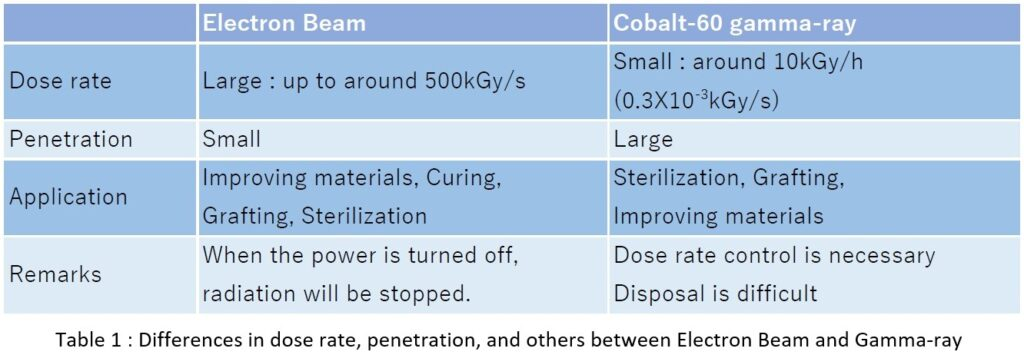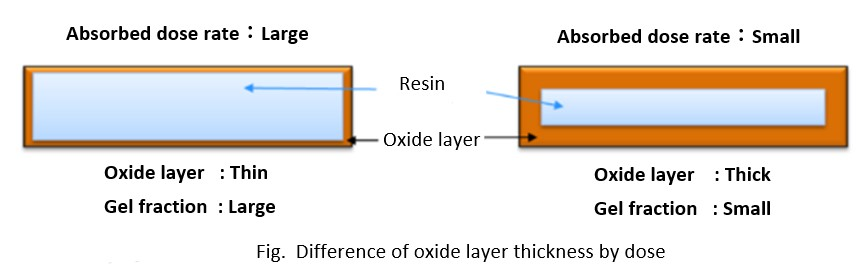## Useful information for Electron Beam

### Dose Rate, Fractional Irradiation, and Their Effects

Posted date:September 01, 2023Last updated:September 01, 2023

In Electron Beam irradiation, the absorbed dose rate is an important parameter for irradiation because it may change the properties of irradiated materials.

This section describes the difference in absorbed dose rate due to the difference in radiation quality, the effects of absorbed dose rate, and the heat generated by electron irradiation and its countermeasures.

#### What is absorbed dose?

Absorbed dose refers to the amount of energy absorbed per unit mass of the irradiated material due to irradiation. Since “Dose” often means “Absorbed dose,” we will refer to “Absorbed dose” as “Dose” in this article. In the International System of Units(SI), the amount of energy absorbed in 1 kg of 1 J (joule) is called 1 Gy (gray), and kGy, 1000 times this amount, is the unit normally used (1,000Gy＝1kGy).

#### What is absorbed dose rate?

The dose absorbed per unit time is called the absorbed dose rate (hereafter simply referred to as “Dose rate”). Therefore, the unit is expressed in kGy/s, etc. For example, the dose rate is equivalent to the work rate (kW) when compared to electricity. When a polymer is irradiated with electron beams at the same dose (kWh) at different dose rates (kW), the physical properties of the polymer may change in different ways.
In electrical terms, if you cook rice in a 1kW rice cooker for 30 minutes and give it 0.5kWh, the rice will cook well, but if you cook rice in this cooker for 5 hours at 0.1kW and give it 0.5kWh, the rice will not cook well and the result will be completely different. The same thing happens with irradiation.

#### Differences in dose rates, etc. between electron beam and gamma-ray

Electron beams can deliver a high dose rate of about 50 kGy per second, while gamma-ray can deliver a dose rate of about 10 kGy per hour, about two orders of magnitude lower.  In other words, electron beams have a low penetrating power and can deliver a large dose to an irradiated material in a short time, while gamma-ray have a high penetrating power and can deliver a small dose to an irradiated material in a short time.
Table 1 summarizes the differences in dose rate, penetration, and others between electron beam and gamma-ray.#### Effects of Dose Rate on Irradiation

Figure 1 shows the oxide layer thickness at different dose rates.
When the dose rate is high, as in the case of electron beams, the reaction rate between the polymer chains and oxygen is large, and irradiation is completed before oxygen diffuses into the resin, so the interior is irradiated in an oxygen-free state and only the surface becomes oxidized. (Figure 1, left)
On the other hand, when the dose rate is low, as in the case of gamma-rays, the reaction rate between the polymer chains and oxygen is slow, so oxygen diffuses into the interior of the resin and the oxidized layer becomes thicker. (Figure 1, right)As explained above, 1 Gy is the amount of 1 J of energy absorbed in 1 kg. 10 kGy is equivalent to about 2.4 cal/g when the dose is converted to calorific value. This is the amount of heat that would be generated if the entire 10 kGy dose were converted into heat for the sample. This is equal to the amount of energy required to raise the temperature of a substance with a specific heat of 1 cal/g-K (e.g., 1 g of water) by about 2.4°C. For polyethylene with a specific heat of 0.5 cal/g-K, the temperature rise is about 4.8°C. When irradiating a large dose such as several hundred kGy, the temperature rise of the sample may be a problem. In such cases, it is effective to divide the irradiation into 5 doses of 100 kGy for 500 kGy irradiation to avoid deformation of the sample due to the rise in temperature. Although the actual dose rate is reduced to 1/5, the effect is negligible.

#### Summary

Depending on the dose rate, the finished product may differ even if the same dose is applied. This is due to oxidative degradation of the material caused by the difference in dose rate. Since there is an effect of temperature rise associated with the dose, care should be taken when irradiating a large dose, such as calculating the estimated temperature rise in advance and using fractional irradiation.

［Inquiry］

NHV Corporation
Please inquire through the inquiry form.

«Previous Next»